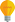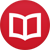# Quantification without variables

by Jakub MarianTip: See my list of theMost Common Mistakes in English. It will teach you how to avoid mis­takes with com­mas, pre­pos­i­tions, ir­reg­u­lar verbs, and much more.

I have written several articles about a way to do classical calculus without using variables, e.g. how to differentiate or integrate functions. I was asked in the comments how to do quantification without variablesyou know, expressions like $∀x > 0$ $∃y > 0: y^2 = x$.

It may seem impossible to do that without using variables, but it turns out it can be done in two different ways, one of which closely resembles the traditional approach while the other looks somewhat strange to an unaccustomed eye.

## The “strange” way

Let’s start with the strange one, so that you get used to working with images of mappings that we will also need later. Let $A$ be a set and $f$ a function. We will use the following notation:

$$f(A) = \{f(x): x ∈ A\}\,.$$

That is, $f(A)$ is the image of $A$ under the mapping $f$. The universal quantifier can be translated as follows:

$$∀x ∈ A: f(x) ∈ B \quad⇔\quad f(A) ⊆ B\,.$$

In particular, we can write

$$∀x ∈ A: f(x) = c \quad⇔\quad f(A) = \{c\}\,.$$

Pretty much any universal quantification you can imagine can be written in this way. The existential quantifier is similar:

$$∃x ∈ A: f(x) ∈ B \quad⇔\quad B ∩ f(A) ≠ ∅\,,$$

in particular

$$∃x ∈ A: f(x) = c \quad⇔\quad c ∈ f(A)\,.$$

How do we combine the two? Suppose you had to rewrite $∀x > 0\,∃y > 0: y^2 = x$ without variableshow would you go about it? You can simply do it in two steps! First, rewrite the inner quantifier:

$$∀x > 0: x ∈ ((0,∞))^2$$

where $(A)^2$ means the image of $A$ under $\id^2$ (the “square function”). Now, “$∀x ∈ A: x ∈$” is the same as “$A ⊆$”, so we can rewrite the last expression as

$$(0,∞) ⊆ ((0,∞))^2\,.$$

Quite neat, isn’t it? It expresses the same idea using a very different kind of thinking. The proof would be quite different, too. Instead of manipulating algebraic expressions, we would prove that $((0,∞))^2 = (0,∞)$ using properties of $\id^2$ (which could be done just by playing around with sets, as all of its properties can be reformulated using the process just described).

## The “normal” way

Another way to look at variables is that they are just projections from a larger space. For example, when working with expressions like $x^2+y^2$, we can think of $x$ and $y$ as functions $ℝ^2 → ℝ$ defined by $x(a,b) = a$ and $y(a,b) = b$. There is a useful notation for working with such functions commonly used in probability theory. Let $R$ be relation (e.g. $<$, $>$, $=$) and $f$ and $g$ functions, then

$$[f\,R\,g] = \{x: f(x)\,R\,g(x)\}\,,$$

for example

$$[{\sin}>0] = ⋃_{k ∈ 2ℤ} (kπ,(k+1)π)\,.$$

So, if $x,y$ are the aforementioned projections, what’s

$$[x^2 + y^2 = 1]\,?$$ The unit circle, of course. Say, we wanted to write down the fact that for each point $(a,b)$ on the unit circle, $|ab| ≤ ÷{1}{2}$. Traditionally, we would write: $$∀x,y ∈ ℝ, x^2 + y^2 = 1: |xy| ≤ ÷{1}{2}$$

(in fact, the “correct” way of writing that would be $∀x ∈ ℝ\,$ $∀y ∈ ℝ:$ $(x^2 + y^2 = 1)$ $⇒$ $(|xy| ≤ ÷{1}{2})$, but I assume everyone understands the shortened version). How to write it without quantification? All we want to express is that the values of $|xy|$ stay within $[0,÷{1}{2}]$ (the closed interval), so we would write

$$|xy|\big([x^2 + y^2 = 1]\big) ⊆ [0,{\textstyle ÷{1}{2}}]\,.$$

If you don’t like the notation for the image of a set, you can also write:

$$[x^2 + y^2 = 1] ⊆ \big[|xy| ≤ {\textstyle ÷{1}{2}}\big]$$

which means that the unit circle lies in the region where $|xy| ≤ ÷{1}{2}$, which is logically equivalent to the previous expression. Nevertheless, there’s a semantic difference between the two; in the first case, we emphasize that the function $|xy|$ has a certain property, whereas in the second case, we emphasize that it is the set $[x^2 + y^2 = 1]$ that has a certain property.

Similarly, going back to the $∀x > 0\,∃y > 0: y^2 = x$ example, understanding the $x$ and $y$ as projections, we can write it as

$$x\big([y > 0] ∩ [y^2 = x]\big) ⊇ (0,∞)\,.$$

It looks crazy at first, but if one understands the underlying logic, it’s actually quite simple: $[y> 0] ∩ [y^2 = x]$ is the set of points $(x,y)$, $y > 0$ that are solutions to $y^2 = x$. Our assertion says that such a solution exists for each $x ∈ (0,∞)$, hence we claim that the projection of $[y > 0] ∩ [y^2 = x]$ to the $x$-axis contains $(0,∞)$.

By the way, I have written several educational ebooks. If you get a copy, you can learn new things and support this website at the same time—why don’t you check them out?